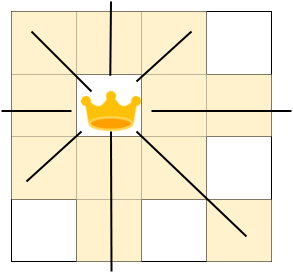# N-Queen

## Introduction

In this article, we will discuss the N queens problem. The N-queen problem is one of the most common and practical examples to understand Backtracking.

## Problem Statement

You are given an integer N. For a given chessboard, find a way to place 'N' queens such that no queen can attack any other queen on the chessboard.

A queen can be attacked when it lies in the same row, column, or the same diagonal as any of the other queens. You have to print one such configuration.

Print a binary matrix as output that has 1s for the cells where queens are placed.

## Backtracking

Cells that can be attacked by a queen is shown below -Let’s take an example to understand the requirement.

Say, N=4. Now, we will try to place the 4 queens such that each of them is safe from every other queen.

In this figure, the red cross mark represents the cells that the queens can attack.

Observe that with this arrangement, we can only place 3 queens, and the 4th queen can't be placed as none of the positions left is safe. So, this is not the desired configuration.

Let’s try to place the queens in some other way -

Hence, the valid configuration is -

0 1 0 0

0 0 0 1

1 0 0 0

0 0 1 0

Note: There can be more than one such valid configuration. In this problem, our goal is just to print one of those.

The key idea is that no two queens can be placed in:

• Same Row
• Same Column
• Same Diagonal

There are rows, so we will place one queen in each row(as no two queens can be in the same column) such that they all are safe from each other.

We will use backtracking to solve the problem. It is a recursive approach in which we place the queen at a safe position and then recur for the remaining queens. If at any step the number of queens to be placed is not zero and there are no safe cells left, then we change the position of the previously placed queen and try another safe position.

Let’s see backtracking the approach step by step -

2. Check the number of queens placed. If it is N, then return true.
3. Check all the columns for the current row one by one.
1. If the cell [row, column] is safe then mark this cell and recursively call the function for the remaining queens to find if it leads to the solution.

If it leads to the solution, return true, else unmark the cell [row, column] ( that is, backtrack) and check for the next column.

1. If the cell [row, column] is not safe, skip the current column and check for the next one.
2. If none of the cells in the current row is safe, then return false.

Output

### Time Complexity -  O(N!)

For the first row, we check N columns; for the second row, we check the N - 1 column and so on. Hence, the time complexity will be N * (N-1) * (N-2) …. i.e. O(N!)

### Space Complexity - O(N^2)

O(N^2), where ‘N’ is the number of queens.

We are using a 2-D array of size N rows and N columns, and also, because of recursion, the recursive stack will have a linear space here. So, the overall space complexity will be O(N^2)

## Optimized Backtracking

Points to consider for optimization -

1. We will not check every cell of the right and left diagonals to determine if a cell is safe.
2. The idea is to use the property of diagonals.
3. For an element with row=i and column=j:
1. The Sum of i and j is constant for each right diagonal.
2. The difference of i and j is constant for each left diagonal.
4. This will reduce the cost of checking if the cell is safe from O(N) to O(1).

Output

### Time Complexity -  O(N!)

O(N!), where ‘N’ is the number of queens and ‘!’ represents factorial.

For the first row, we check ‘N’ columns, for the second row, we check the N - 1 column and so on. Hence time complexity will be N * (N-1) * (N-2) …. i.e. N!

### Space Complexity - O(N^2)

O(N^2), where ‘N’ is the number of queens as we are using a 2-D array having rows and columns.

1.  What is backtracking?

Backtracking is an algorithmic technique to solve the problems recursively and remove those solutions that do not satisfy the problem constraints at any point in time.

2.  When is backtracking used?

It is generally used in problems where you have multiple options and after choosing one option, you have a set of new options hence recursion. The process continues until a final state or the desired state is reached.
3.  In chess, in how many directions a queen can attack?

A queen can attack in 3 directions - horizontally, vertically and diagonally.
4. What is the worst-case time complexity of the brute force approach to solving the N queen problem?

The worst-case “brute force” solution for the N-queens puzzle has an O(N^N) time complexity. This means it will look through every position on an NxN board, times, for queens.

## Key Takeaways

In this article, we learnt to solve the famous N queen problem using backtracking. If you want to master backtracking, learn Backtracking here. We also analyzed the time and space complexity of the approach along with its implementation. Further, we also saw the optimized backtracking approach.

Do check out Rat in MazeThe Knight’s tour problems which are also based on backtracking.

Are you planning to ace the interviews of reputed product-based companies like Amazon, Google, Microsoft, and more? Attempt our Online Mock Test Series on CodeStudio now!